## Introduction

The incentive for this tutorial arose when a student asked about the error in a receiver function. The teleseismic P-wave receiver function is a filter which converts the vertical component of the P wave to the radial component. Typically this filter is defined as that which converts the observed P-wave on the vertical component to a low-pass filtered P-wave on the radial component. This low pass operation is usually defined in terms of a Gaussian function

H(f) = exp [ -  ( π f / α ) 2 ]

If the filter determined from the data confidently predicts the low-pass filtered radial component, and if the filter satisfies elementary elastic wave theory, then the resultant receiver function can be used constrain Earth structure near the seismograph station.

The purpose of this tutorial is to use the codes of Computer Programs in Seismology to synthesize an observed teleseismic P-wave signal, to add a realistic ground noise, and then to compare the receiver functions based on noisy data to that based on noise free data.

This exercise is contained in the gzip'd tar file RFTNNOISE.tgz

          gunzip -c RFTNNOISE.tgz | tar xf -
cd RFTNNOISE.dist/src
make all
cd ..

This procedure creates the directory structure:
          RFTNNOISE.dist/
RFTNNOISE.dist/DOCLEANUP              Script to clean up temporary files
RFTNNOISE.dist/DOIT			Simulation script
RFTNNOISE.dist/DOPLOT                 Script to create PNG graphics
RFTNNOISE.dist/Models/                Directory having models for making synthetics
RFTNNOISE.dist/src/
RFTNNOISE.dist/src/Makefile
RFTNNOISE.dist/src/noisemodel.h
RFTNNOISE.dist/src/sacnoise.c
RFTNNOISE.dist/src/sacsubc.c
RFTNNOISE.dist/src/sacsubc.h
RFTNNOISE.dist/Models/CUS.mod
RFTNNOISE.dist/Models/tak135sph.mod

As a result of the make all the executable sacnoise is created in the src directory.

## DOIT

DOIT  is the driving script for the simulations. It is bash shell script with all controls at the top of the file. To keep the code manageable, the bash functions are used. These appear first in the file. The logic of the simulation is incorporated in these lines:

############################################################################
#    main processing
############################################################################

#####
#   clean up from previous runs
#####
cleanup
#####
#    initialize the random number sequence
#####
init
#####
#    make the teleseism signal
#    and then get the receiver function from the noisefree signal
#####
maketeleseism
dorftnnonoise
#####
#    add noise to the Z and R components for the teleseismic P wave
#####
SIM=00
do
SIM=echo $SIM | awk '{printf "%3.3d",$1 + 1 }' 
domakenoise
dorftnnoise
done < surd.tmp
#####
#     now stack the noisy P signals to see if they are improved
#     stack the RFTNs from the noisy signal
#####
dostack

The comments indicate what each function does. init() creates  NSIM random numbers between 0 and 9999 in the file surd.tmp, with one entry per line. maketeleseism uses hudson96 to make the teleseismic P wave signal on the Z and R components. The amplitude of the synthetic depends on Mw and on a source duration which is a function of Mw.  dorftnnonoise creates the receiver functions for α = 0.5, 1.0 and 2.5.
domakenoise     creates the noise for the Z and R components. The PVAL , which is 0 ≤ PVAL ≤ 1
indicates the level of gound noise with the lower limit of 0 corresponding to the NLNM (New Low Noise Model) and   the upper limit of 1 corresponding to the NHNM (New High Noise Model). There are three options to the creation of the noise. For NOISE=0 the noise sequences for the Z and R are derived from different random number surds. For NOISE=1 the noise for the R component is the same as that on the vertical. For NOISE=2 the noise on the R component is the negative of that on the vertical component. Finally for NOISE=3 the noise on the R component is the Hilbert transform of that on the vertical. The last 3 options simulate  P-wave, SV-wave and Rayleigh wave noise, respectively when comparing the Z and R components.  Finally the noise for each component is added to the teleseismic signal for that component, and the receiver functions are estimated.

## Simulations

Figure 1 gives the ground velocity (m/s) for an Mw=6.0 soruce at a depth of 20 km at a distance of 40 degrees from the receiver. The receiver is at an azimuth of 45 degrees from the source. The fault plane parameters are strike= 80, dip = 80 and rake = 10. In this figure T.Z is the vertical component motion and T.R is the radial motion, 1.0.sac.1354 is the ground noise in m/s that is used with the he Z component, and 2.0.sac.1354 is the ground noise for the radial component. For this simulation NOISE=1 so that the noise on the two components is the same.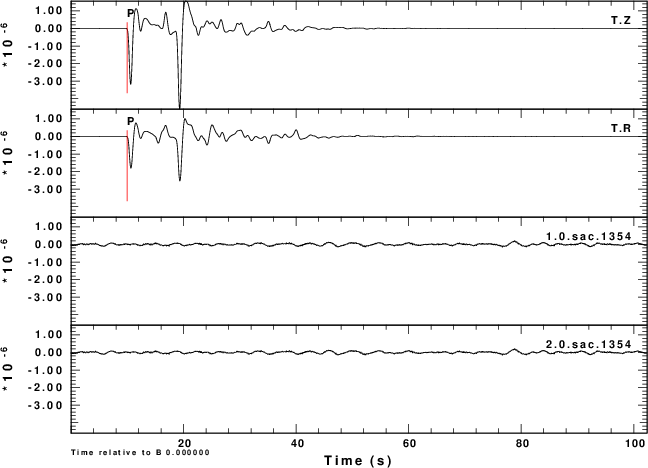Fig. 1. Teleseismic signal and noise

Figure 2 shows the simulated noisy Z and R waveforms for each of the of the nine noise sequences and for the four choices of the NOISE parameter.
 Fig. 2. Simulated noisy signals Simulation Z component R component NOISE=0 R and Z noise are independent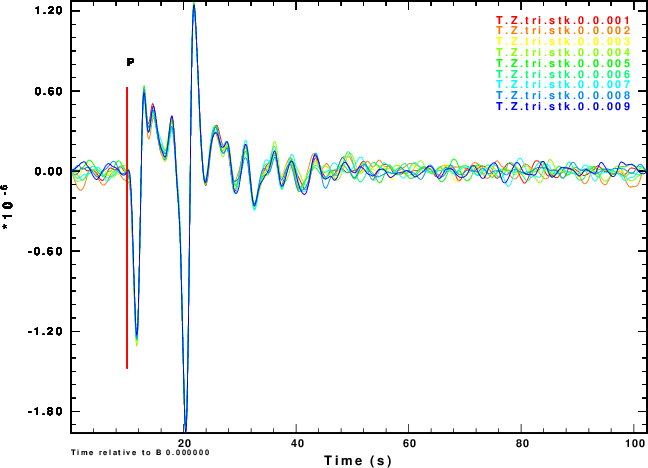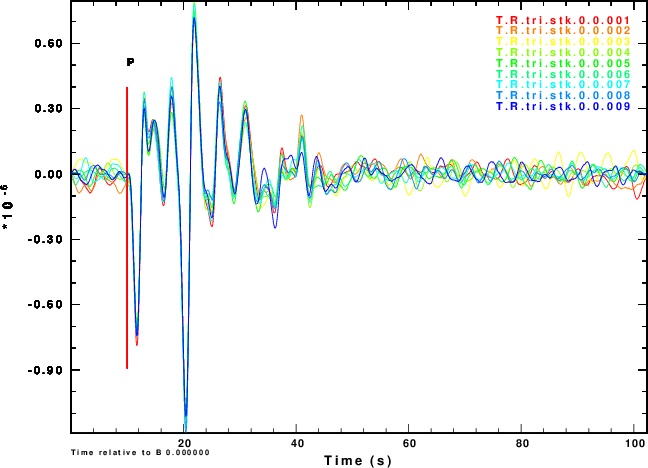NOISE=1 R noise is Z noise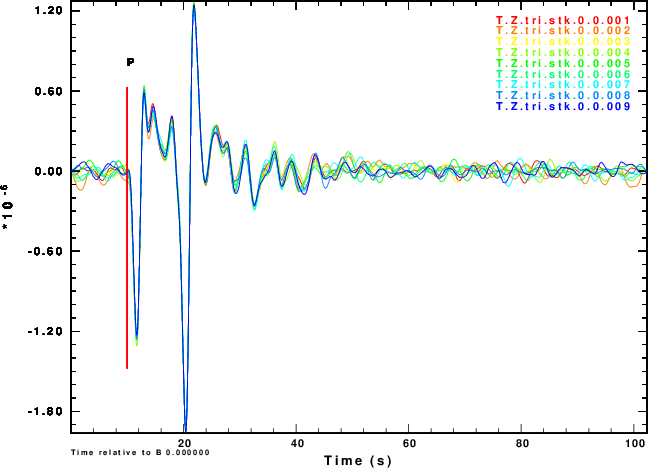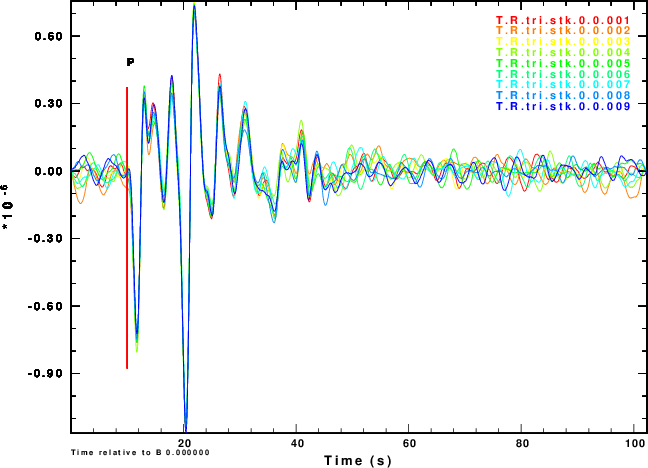NOISE=2 R noise is negative of Z noise≈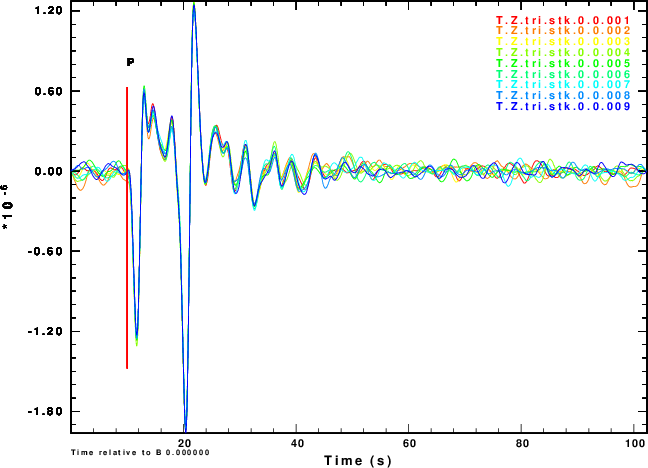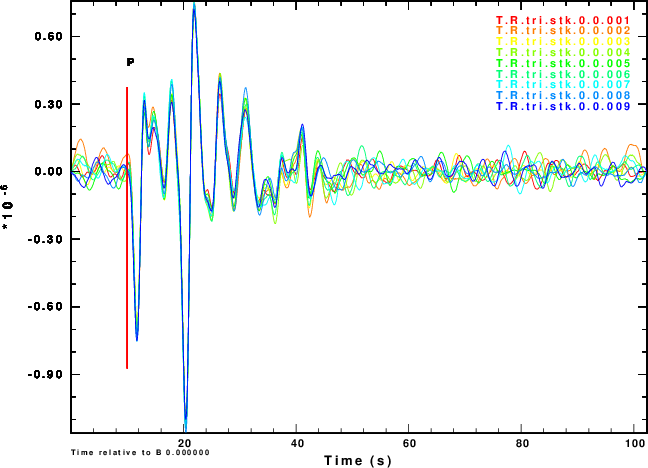NOISE=3 R noise is Hilbert transform of Z noise≈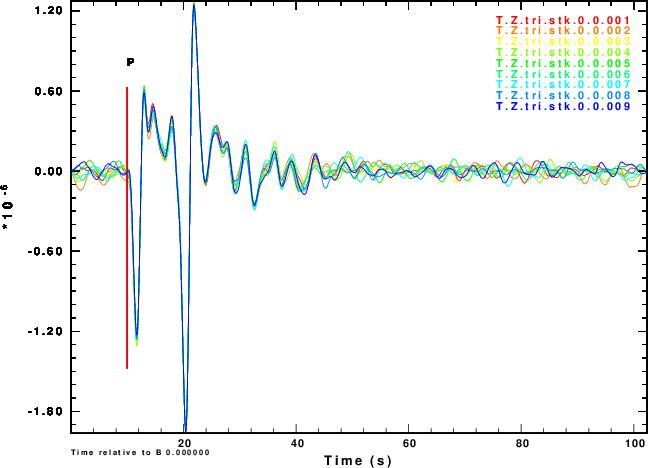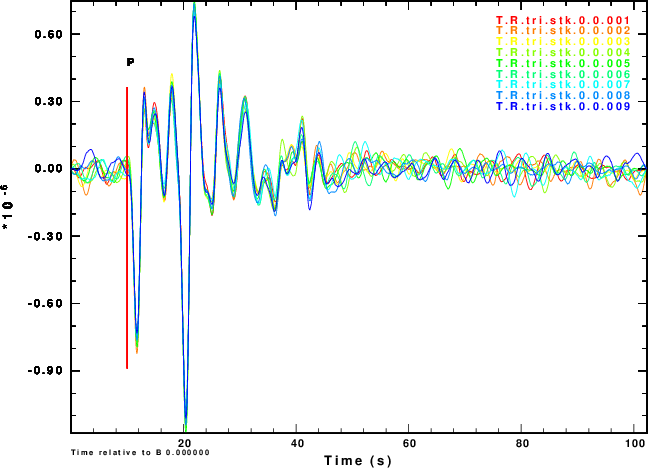To see how stacking might improve the signals, we can just stack the individual traces. The results are shown in Figure 3. This stacking procedure is normally not done with real data, but could be done.
 Fig. 3. Stacked teleseismic signals Simulation Normalized stack NOISE=0 R and Z noise are independent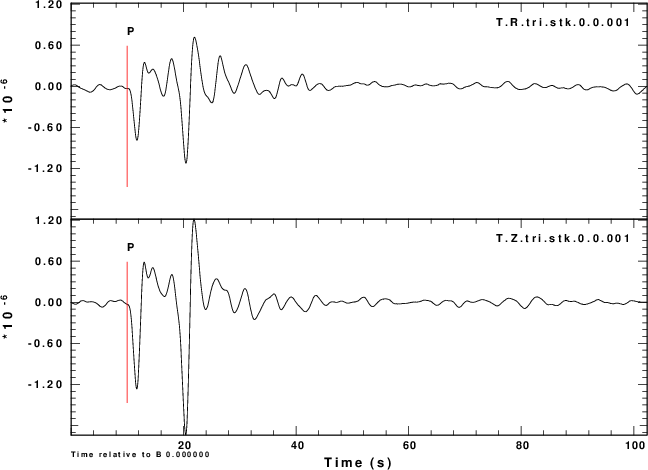NOISE=1 R noise is Z noise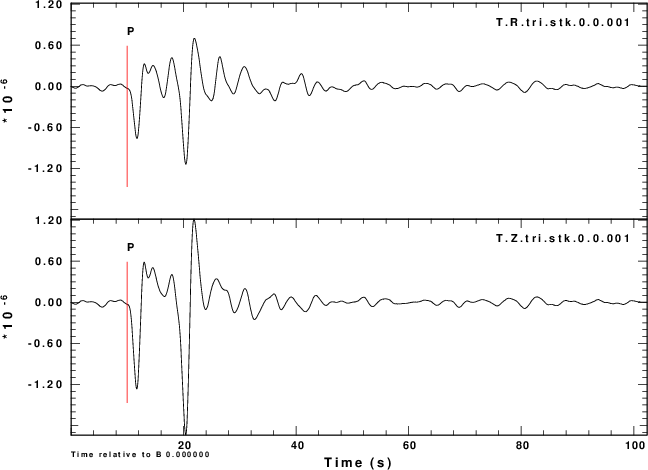NOISE=2 R noise is negative of Z noise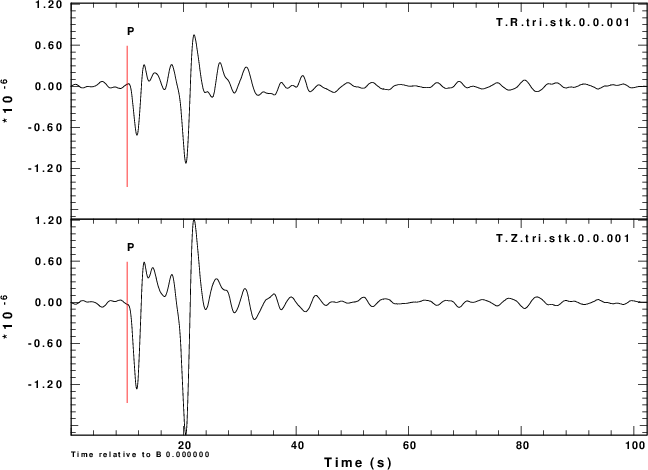NOISE=3 R noise is Hilbert transform of Z noise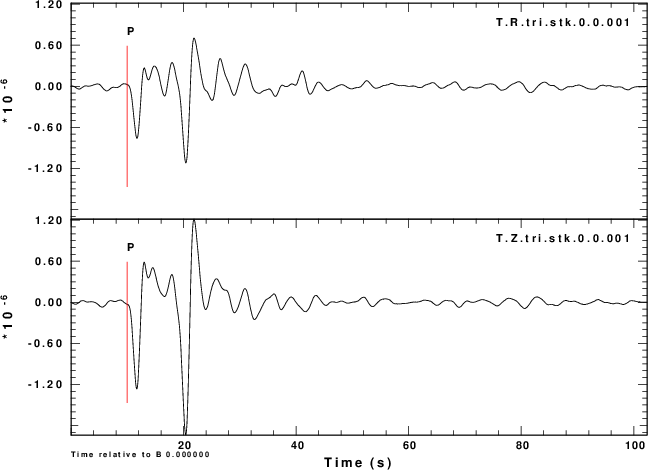The next exercise is to compute the receiver functions using the iterative deconvolution program saciterd of Ligorria and Ammon. These are shown in Figure 4 for the three values of $\alpha = 2.5$.

 Simulation α = 0.5 α = 1.0 α =2.5 NOISE=0 R and Z noise are independent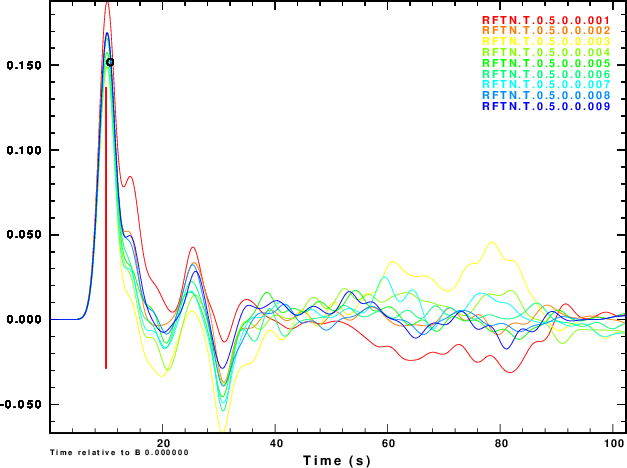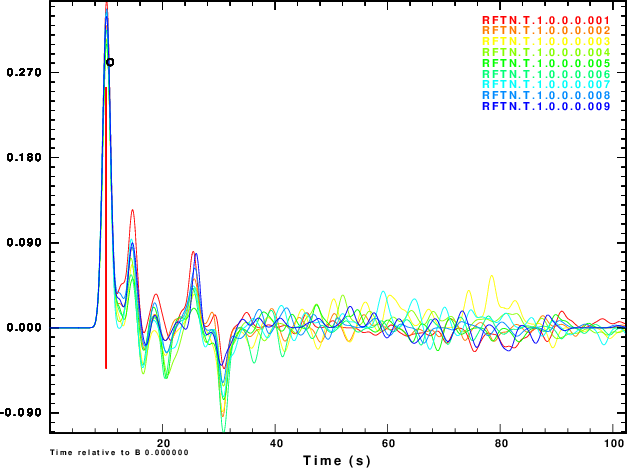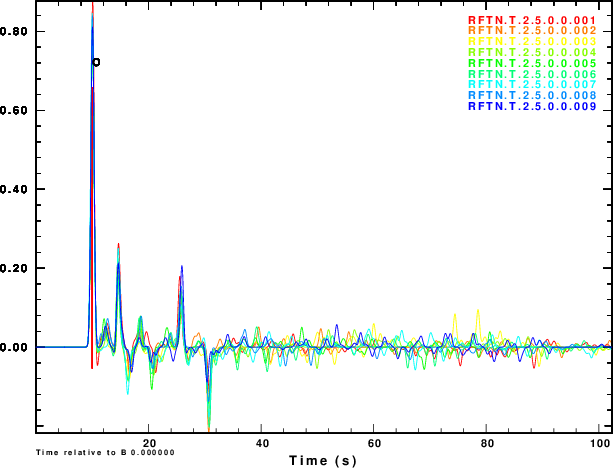NOISE=1 R noise is Z noise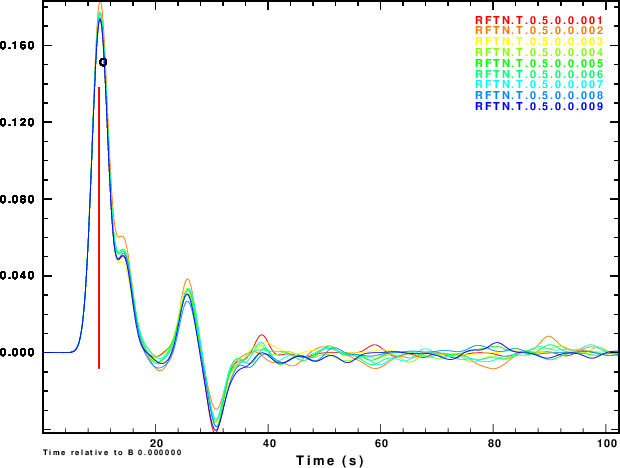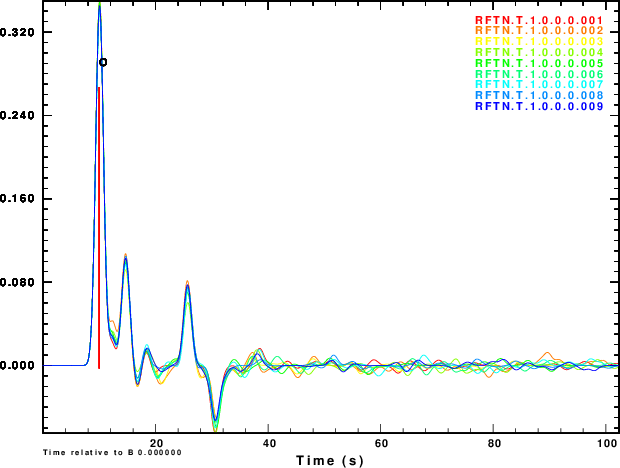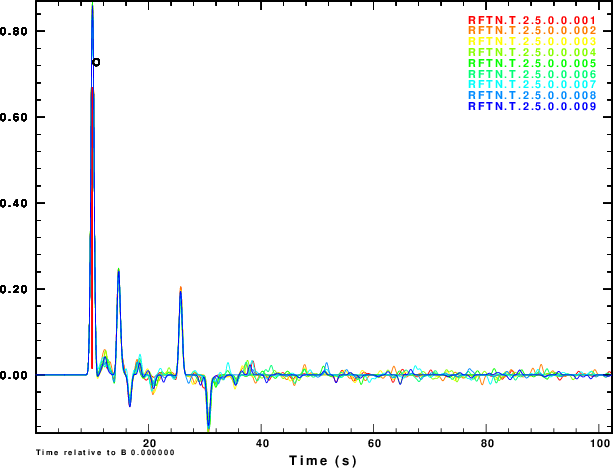NOISE=2 R noise is negative of Z noise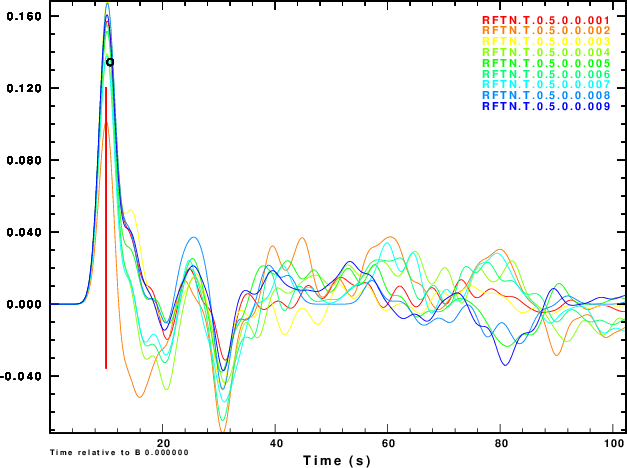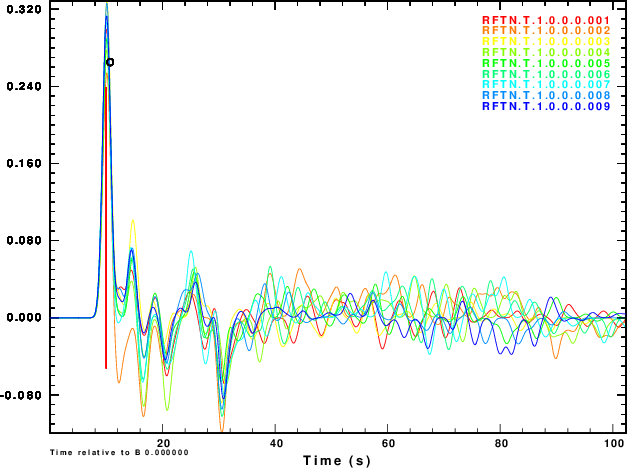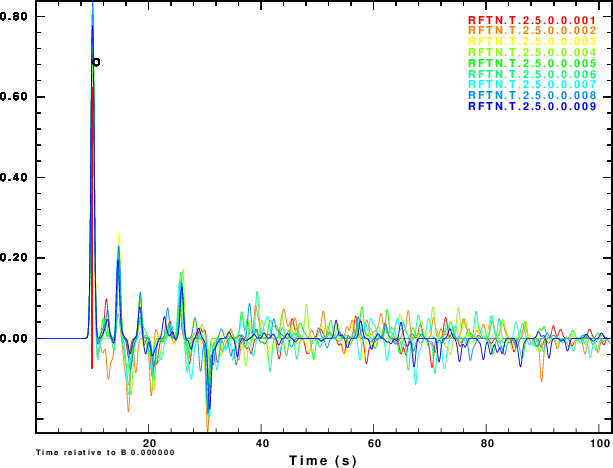NOISE=3 R noise is Hilbert transform of Z noise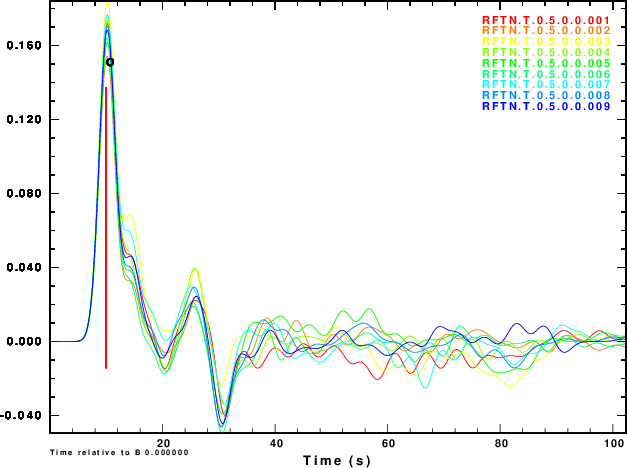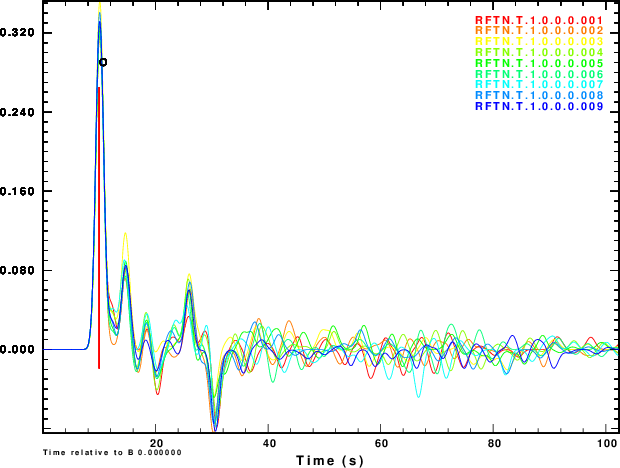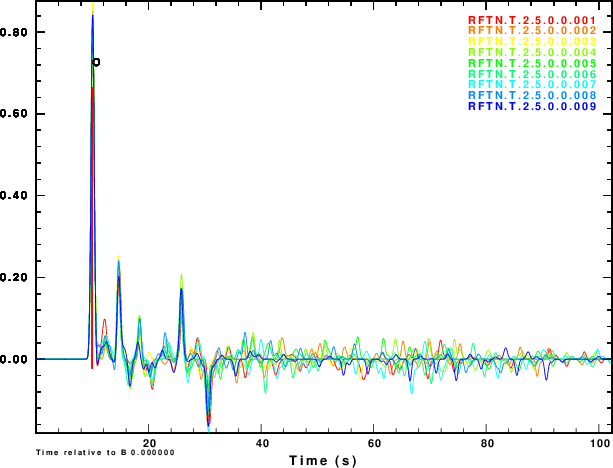Fig. 4. Receiver functions for each of the noisy observations for the three α values.

Finally we compare normalized stacked receiver functions to the those of the noise-free signals in Figure 5.

 Fig. 5. Comparison of the normalized stacked receiver functions (blue) to the noise-free receiver function (red) for the observations for the three α values. Simulation α = 0.5 α = 1.0 α =2.5 NOISE=0 R and Z noise are independent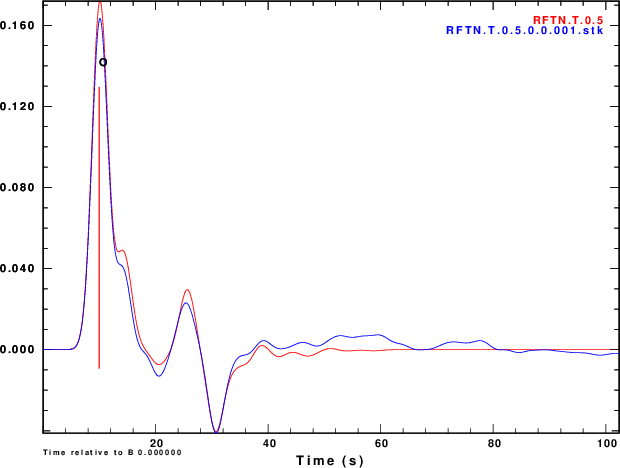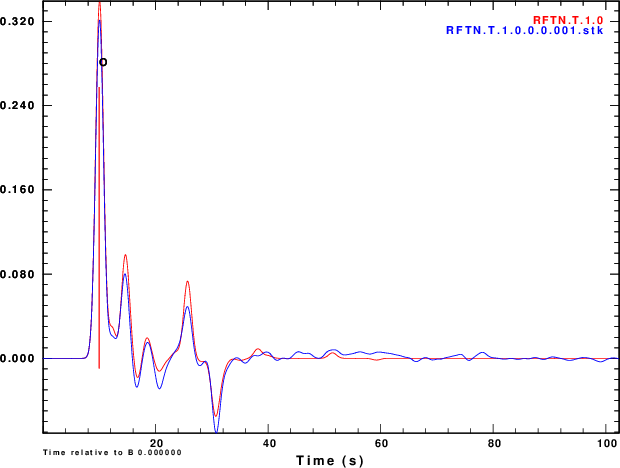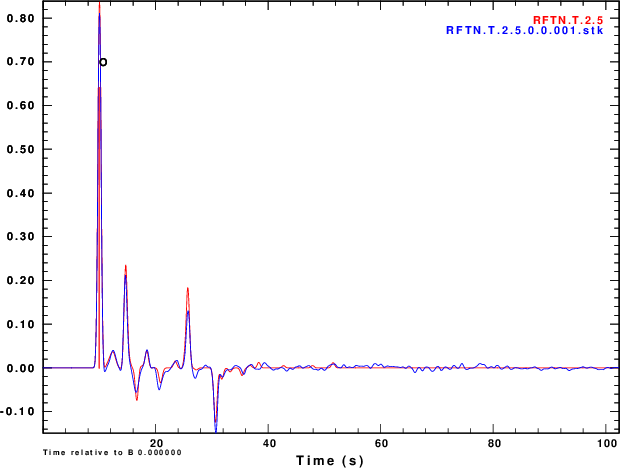NOISE=1 R noise is Z noise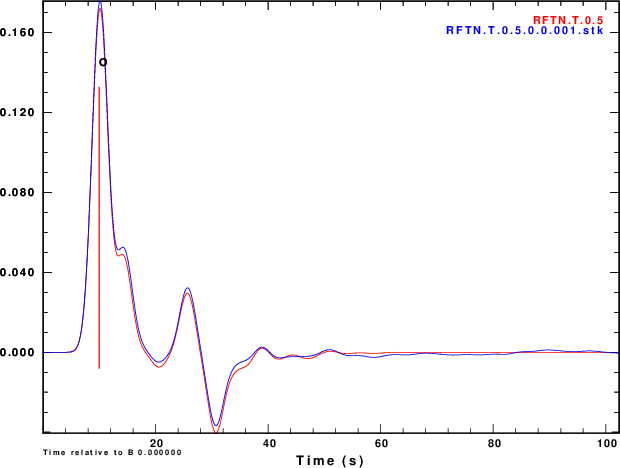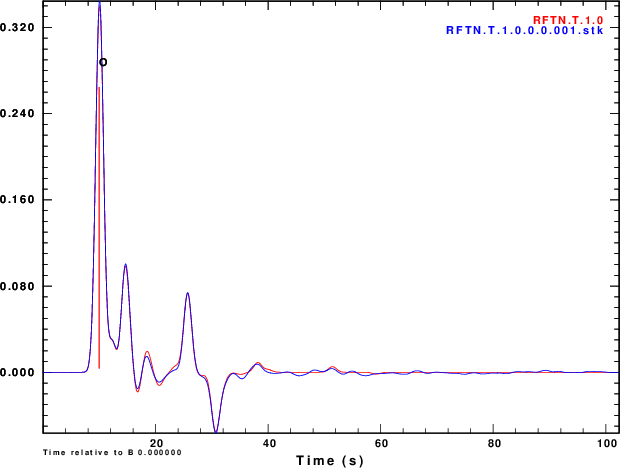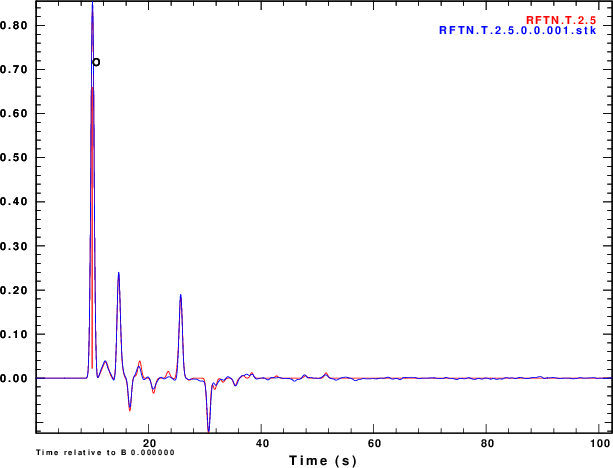NOISE=2 R noise is negative of Z noise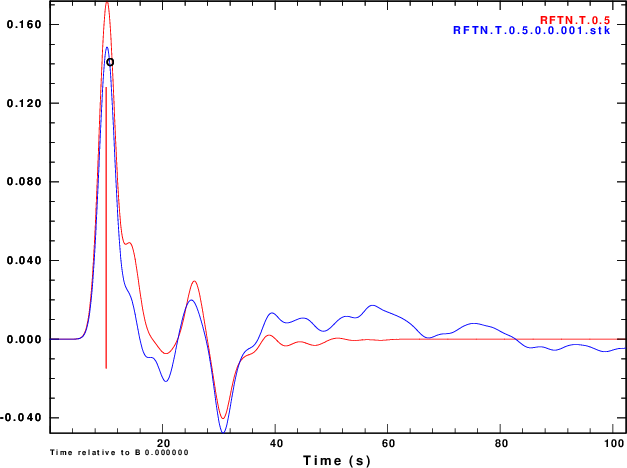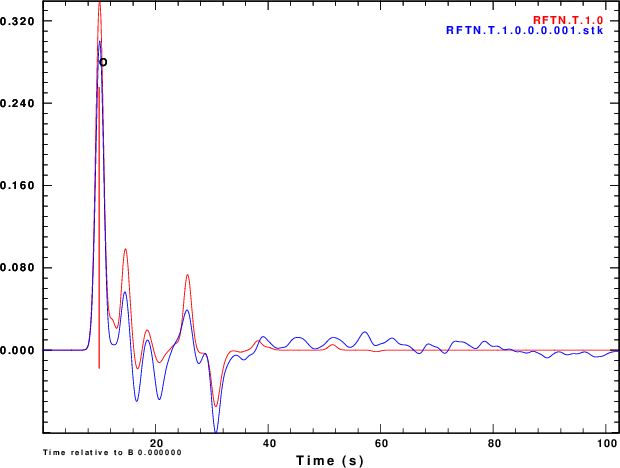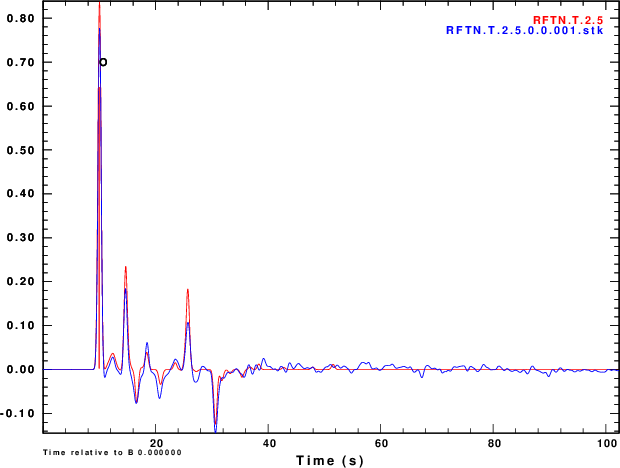NOISE=3 R noise is Hilbert transform of Z noise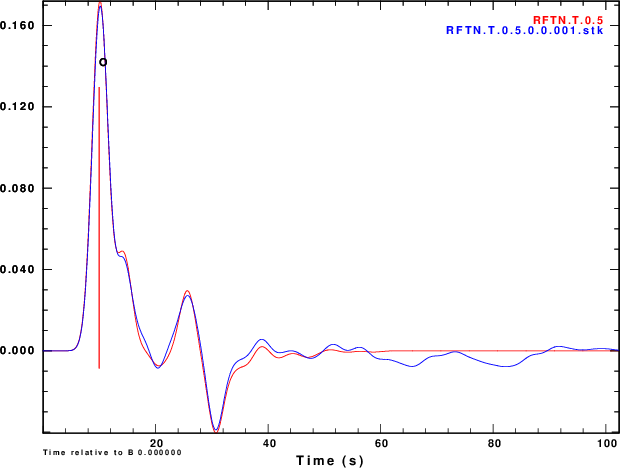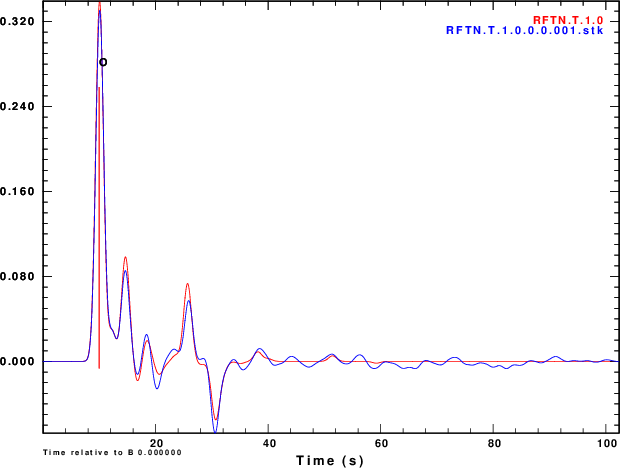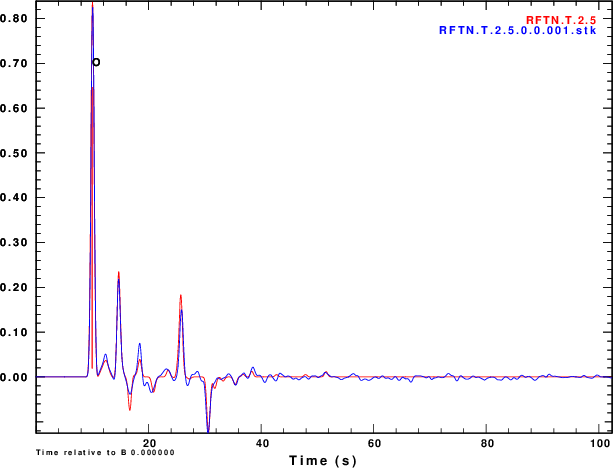## Discussion

Several objectives of this exercise have been met. First an example is provided of how to add realistic noise to synthetics and then to processess thos synthetics. In this case we focus on teleseismic P-wave receiver functions.

Figure 4 shows that noise can affect the determinationof the receiver function. The effect is strongest for α = 0.5 and less noticable for α = 2.5. So waht use the lower value. Well other simulations of the effect of deep sediments at the reciever indicate that these are not as storng for the lower value of α. One way of thinking about this is that the smaller alpha emphasizes the lower frequencies,  for which the Earth starts to look mmore like a halfspace.

We slao see that the effect of the noise in the inividual receiver functions of Figure 4 and on the stacks of Figure 5 are not so bad if the nosie is the same on the vertical and radial components or if the two noises are holber transforms of each other. Ammon (199X) showed the the First bump of the receiver function descroibes the conversion of incident P on the vertical to the radial, while later features refpresent the effect of the conversion of the S wave on the vertical to that on the radial. For NOISE=1, he motion is P-wave motion.  For NOISE=3 the motion may be Rayleigh like. Now with real data, the direction of plane wave nosie is no know, and even though it may be due to a P-wave, if it is incident from a direction other than the back azimuth, then there will be some of the NOISE=2 in the data.

Although the amplitudes are sensitive to the noise, the timing of major pahses is not as sensitive.

I would expect that the results would be worse, if the PVAL is a more reasonable value, such as 0.2 for an Mw=6.  This simulation reflects real expeience, in that one needs a lot of large earthquake eata to make a good receiver function.

Last changed December 16, 2017